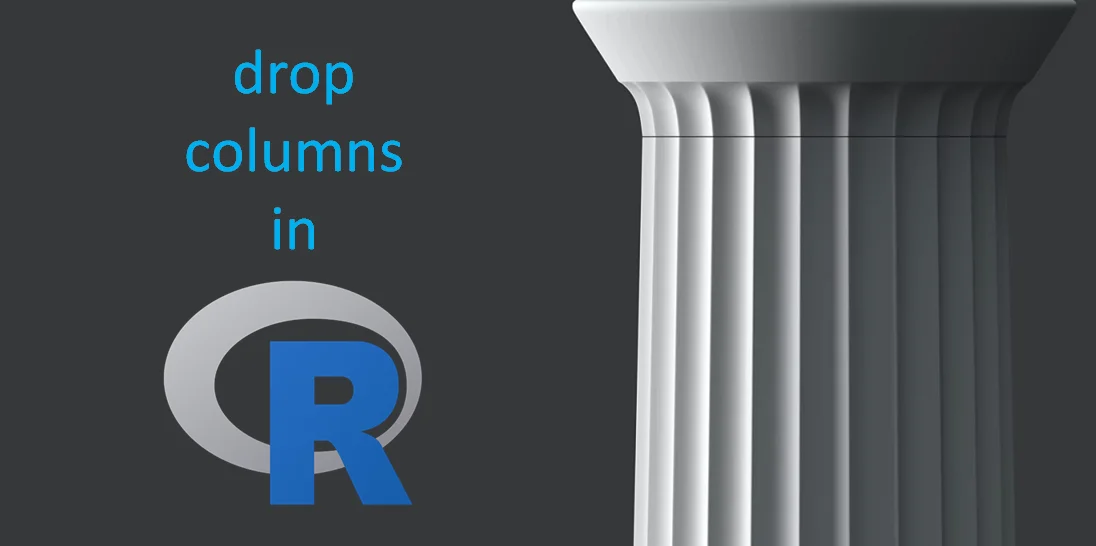# How to quickly drop columns in R in data frame

Here are multiple ways how to drop one or multiple columns in the R data frame. You can use R base functionality or package like dplyr, but it is not the most time-consuming operation.
By knowing how to do that in different ways, you can choose a suitable approach.

## Quickly drop columns in base R

Here is one of my favorite ways to drop columns in R if there is a small amount. As you can see, there are no additional functions involved. Choose a column that you want to drop and replace it with NA.

```df <- iris
names(df)
# "Sepal.Length" "Sepal.Width"  "Petal.Length" "Petal.Width"  "Species"

df\$Species <- NULL
names(df)
# "Sepal.Length" "Sepal.Width"  "Petal.Length" "Petal.Width"```

You can use the same approach to remove multiple columns at the same time.

```df <- iris
df\$Species <- df\$Sepal.Width <- NULL

names(df)
# "Sepal.Length" "Petal.Length" "Petal.Width"```

## Drop R data frame columns by column index number or range

Here is how to locate data frame columns by using index numbers or a certain range and drop them. Watch out for situations when a position is changing. The following methods that involve column names might be a safer approach.

```df <- iris
df <- df[-c(2, 3)]
names(df)
# "Sepal.Length" "Petal.Width"  "Species"

#range of columns
df <- iris
df <- df[-c(2:4)]
names(df)
# "Sepal.Length" "Species"```

## Drop columns in R by the list of column names

Let’s say you have a list of column names that you want to remove from a data frame. Here is how to use them in that scenario.

```df <- iris

rem <- c("Species"
, "Sepal.Width"
, "Petal.Width")

df <- df[!(names(df) %in% rem)]
names(df)

# "Sepal.Length" "Petal.Length"```

## Remove columns by using a keyword

If you have a keyword that defines which of the data frame columns should be removed, then here is how to use that. If you have a specific situation and you should use a part of the column name that is at the beginning or the end, then take a look at the following methods that involve dplyr.

```df <- iris
df <- df[!grepl('Width', names(df))]
names(df)

# "Sepal.Length" "Petal.Length" "Species"```

## Drop unnecessary data frame columns with dplyr

Select function from the dplyr package lets you choose a necessary column. Add a minus sign to the column name in the dplyr select function that you want to drop from the data frame.

```names(iris)
# "Sepal.Length" "Sepal.Width"  "Petal.Length" "Petal.Width"  "Species"

require(dplyr)
iris %>% select(-Species) %>% names()
# "Sepal.Length" "Sepal.Width"  "Petal.Length" "Petal.Width"```

It can also be done more advanced way. If you have a keyword, you can use the dplyr function contains and drop all the columns with names that include that.

```iris %>% select(-contains('Width')) %>% names()
# "Sepal.Length" "Petal.Length" "Species"```

That is one of my top 10 favorite dplyr tips and tricks and if you like this one, then take a look at others.

Take a look at other available options that are available in the dplyr package. There are also functions like starts_with if you should drop columns by the beginning of names or ends_with if you should drop columns by the end of names.

```iris %>% select(-ends_with('Width')) %>% names()
# "Sepal.Length" "Petal.Length" "Species"```

## You might be interested

If you like to improve your work with R, then here are my favorite RStudio tips and tricks.

Categories R